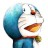# 杰拉斯的博客

## 探寻C++最快的读取文件的方案杰拉斯 | 时间：2012-03-31, Sat | 8,330 views

```#include <ctime>
int main()
{
int start = clock();
//DO SOMETHING
printf("%.3lf\n",double(clock()-start)/CLOCKS_PER_SEC);
}
```

```const int MAXN = 10000000;
int numbers[MAXN];
{
freopen("data.txt","r",stdin);
for (int i=0;i<MAXN;i++)
scanf("%d",&numbers[i]);
}
```

```const int MAXN = 10000000;
int numbers[MAXN];
{
freopen("data.txt","r",stdin);
for (int i=0;i<MAXN;i++)
std::cin >> numbers[i];
}
```

```const int MAXN = 10000000;
int numbers[MAXN];
{
freopen("data.txt","r",stdin);
std::ios::sync_with_stdio(false);
for (int i=0;i<MAXN;i++)
std::cin >> numbers[i];
}
```

```const int MAXS = 60*1024*1024;
char buf[MAXS];
void analyse(char *buf,int len = MAXS)
{
int i;
numbers[i=0]=0;
for (char *p=buf;*p && p-buf<len;p++)
if (*p == ' ')
numbers[++i]=0;
else
numbers[i] = numbers[i] * 10 + *p - '0';
}
```

```const int MAXN = 10000000;
const int MAXS = 60*1024*1024;
int numbers[MAXN];
char buf[MAXS];
{
freopen("data.txt","rb",stdin);
buf[len] = '\0';
analyse(buf,len);
}
```

```const int MAXN = 10000000;
const int MAXS = 60*1024*1024;
int numbers[MAXN];
char buf[MAXS];
{
int fd = open("data.txt",O_RDONLY);
buf[len] = '\0';
analyse(buf,len);
}
```

```const int MAXN = 10000000;
const int MAXS = 60*1024*1024;
int numbers[MAXN];
char buf[MAXS];
void mmap_analyse()
{
int fd = open("data.txt",O_RDONLY);
int len = lseek(fd,0,SEEK_END);
char *mbuf = (char *) mmap(NULL,len,PROT_READ,MAP_PRIVATE,fd,0);
analyse(mbuf,len);
}
```

```const
MAXN = 10000000;
var
numbers :array[0..MAXN] of longint;
i :longint;
begin
assign(input,'data.txt');
reset(input);
for i:=0 to MAXN do
end.
```

scanf 2.010 3.704 3.425
cin 6.380 64.003 19.208
cin取消同步 2.050 6.004 19.616
mmap 0.250 不支持 不支持

1. Linux平台上运行程序普遍比Windows上快。
2. Windows下VC编译的程序一般运行比MINGW（MINimal Gcc for Windows）快。
3. VC对cin取消同步与否不敏感，前后效率相同。反过来MINGW则非常敏感，前后效率相差8倍。
5. Pascal程序运行速度实在令人不敢恭维。

#### 2 条评论 »

1.maxwell

根本原因是因为硬盘和内存的读写特性不同。硬盘的读写是按“块”的。测试的很好，有数据，有对比

2.luyao

windos的是debug的还是release的？